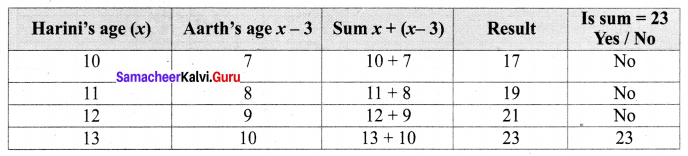# Samacheer Kalvi 6th Maths Solutions Term 1 Chapter 2 Introduction to Algebra Additional Questions

## Tamilnadu Samacheer Kalvi 6th Maths Solutions Term 1 Chapter 2 Introduction to Algebra AdditionalQuestions

Question 1.
Solution:
0

Question 2.
Multiplicative identity ____
Solution:
1

Question 3.
Express to an algebraic statement.
(i) ‘t’ is added to 100
(ii) 4 less to 9 times of y.
Solution:
(i) t + 100
(ii) 9y – 4

Question 4.
Find the rule which gives the number of sticks in the following pattern.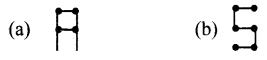Solution:
Let ‘x’ be the no. of R’s formed.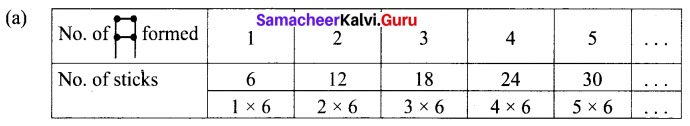The rule is 6x.
Let y ’ be the no. of S’s formed.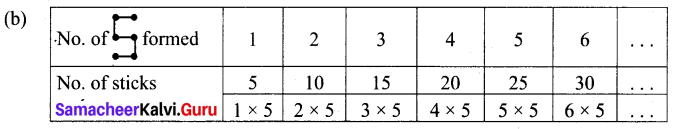The rule is 5y.

##Question 5.
How old was Suja 6 years from now?
Solution:
Let Suja’s present age be ‘a’ years.
6 years from now Suja will be (a + 6) years old.

Question 6.
Price of Apple per kg is ₹ 50 more than price of orange per kg. What is the cost of Apple per kg?
Solution:
Let the price of orange be ₹ b
Price of Apple will be ₹ (b + 50)

Question 7.
Given ‘n’ students like ice cream. What may 2n show?
Solution:
2n shows double the number of students who like ice cream.

Question 8.
Price of oil per litre is ₹ 5 more than three times the price of cool drinks ₹ ‘p’ Express algebraically.
Solution:
Price of cool drinks per kg = ₹ p
Three times = 3p
5 Rs. more = 3p + 5
Price of oil per kg = ₹ (3p + 5)

Question 9.
Complete the table and by inspection of the table find the value of m when m + 10 = 16.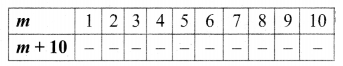Solution: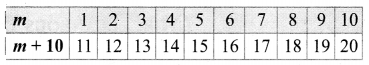From the table m+ 10 = 16 when m = 6.

##Question 10.
Express algebraically (a) y divided by r (b) double times x is subtracted from 10
Solution:
(a) $$\frac{y}{r}$$
(b) 10 – 2x

Question 11.
Give verbal expression of
(a) 7x + 18
(b) $$\frac{4 x}{3}$$
Solution:
(a) 18 added to 7 times x
(b) 4 times x divided by 3.

Question 12.
Rajini’s Father’s age is 5 years more than 3 times Rajini’s age. What is her father’s age?
Solution:
3x + 5

Question 13.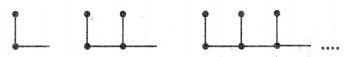Find the rule for the above pattern.
Solution:
2p

Question 14.
Prepare a table for 3x + 10. From the table find the value of x when 3x + 10 = 25.
Solution:
5

Question 15.
Complete the table and find the solution of the equation $$\frac{z}{3}=4$$ using the table.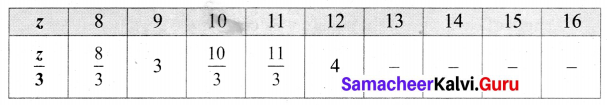Solution: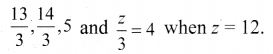Question 16.
Form the expression for which Ramu is 3 years younger than Mathu.
Solution:
m – 3

##Question 17.
A tap is to be pasted along the edges of a square shaped gift box. Its length is 4 cm. What is the length of tap needed for one side.
Solution:
$$\frac{4 p}{4}=p$$

Question 18.
The value of y in 7y – 20 = 99.
Solution:
y = 17

Question 19.
Nine added to two times x gives 301. Find the value of x.
Solution:
x = 146

Question 20.
Aarthi is 3 years younger to Harini. If the sum of their ages is 23, how old is Harini?
Solution:
Let Harini’s age be x years
Aarthi’s age is x – 3 years
Given sum of their ages is 23.
i.e., x + (x – 3) = 23